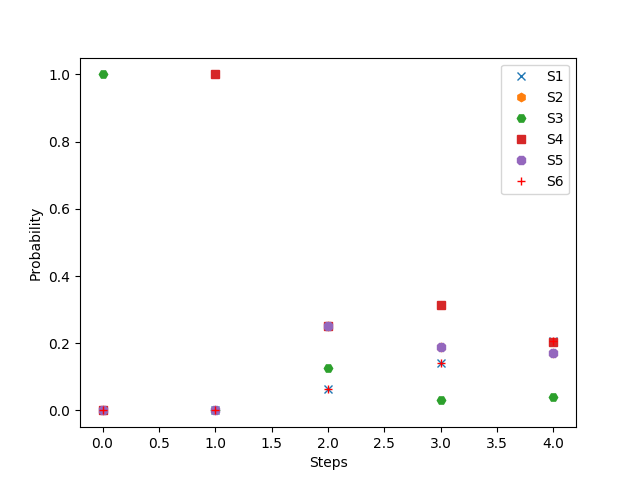# 举例

S1 = {AA, AA},
S2 = {AA, Aa}
S3 = {AA, aa}
S4 = {Aa, Aa}
S5 = {Aa, aa}
S6 = {aa, aa}.

StateNext(S1)Next(S2)Next(S3)Next(S4)Next(S5)Next(S6)
Current(S1) 100000
Current(S2) 1/41/201/400
Current(S3) 000100
Current(S4) 1/161/41/81/41/41/16
Current(S5) 0001/41/21/4
Current(S6) 000001

## 应用马尔可夫链

#!/usr/bin/env python

import numpy as np
from matplotlib import pyplot

# state transition matrix
P = np.matrix([[1., 0., 0., 0., 0., 0.],
[1./4, 1./2, 0., 1./4, 0., 0.],
[0., 0., 0., 1., 0., 0.],
[1./16, 1./4, 1./8, 1./4, 1./4, 1./16],
[0., 0., 0., 1./4, 1./2, 1./4],
[0., 0., 0., 0., 0., 1.]])

# Init state
v = np.matrix([[0, 0, 1, 0, 0, 0]])

# Get the data
plot_data = []
for step in range(5):
result = v * P**step
plot_data.append(np.array(result).flatten())

# Convert the data format
plot_data = np.array(plot_data)

# Create the plot
pyplot.figure(1)
pyplot.xlabel('Steps')
pyplot.ylabel('Probability')
lines = []
for i, shape in zip(range(6), ['x', 'h', 'H', 's', '8', 'r+']):
line, = pyplot.plot(plot_data[:, i], shape, label="S%i" % (i+1))
lines.append(line)
pyplot.legend(handles=lines, loc=1)
pyplot.show()

• 运行：
pip install numpy matplotlib
python markov_chain_example.py

• 结果：## 结论

### 矩阵运算

result = v * P**step


# Refers

Python Markov Chain Packages

Chatopera 联合创始人 & CEO，运营聊天机器人平台 https://bot.chatopera.com，让聊天机器人上线！2015年开始探索聊天机器人的商业应用，实现基于自然语言交互的流程引擎、语音识别、自然语言理解，2018年出版《智能问答与深度学习》一书。10-07153
04-221万+
09-11731
10-20142
10-1988
10-1663
10-16126
10-15607
10-12458
10-1235